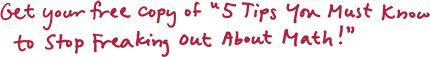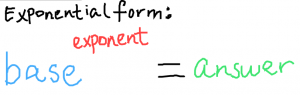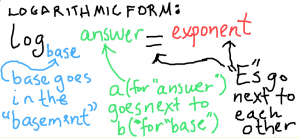Rebecca Zook - Math Tutoring OnlineCall me free of charge to discuss your situation, and we'll see if I can help.

617-888-0160

An Easy Way to Remember How Logarithmic Notation Works

Saturday, April 10th, 2010

Here’s a way my students and I developed to help remember what goes where in logarithmic form.

Many of my students have found this really helps them remember logarithmic notation!!While this memory device is no substitute for understanding conceptually how logarithms work, it is very useful to be able to remember how to “rearrange the furniture” to change an exponential equation into a logarithmic equation.

And speaking of logarithms, I also highly recommend Kate Nowak‘s post on how to introduce logarithms without freaking students out.

Maria Droujkova of Natural Math also has a great post on how you can use family trees to demonstrate how logarithms work.